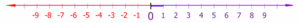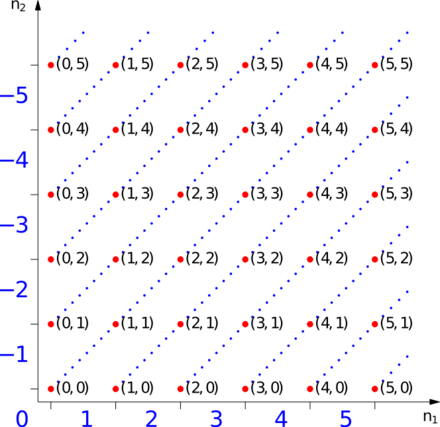# Integer

﻿
IntegerSymbol often used to denote the set of integers

The integers (from the Latin integer, literally "untouched", hence "whole": the word entire comes from the same origin, but via French) are formed by the natural numbers (including 0) (0, 1, 2, 3, ...) together with the negatives of the non-zero natural numbers (−1, −2, −3, ...).They are known as Positive and Negative Integers respectively. Viewed as a subset of the real numbers, they are numbers that can be written without a fractional or decimal component, and fall within the set {..., −2, −1, 0, 1, 2, ...}. For example, 21, 4, and −2048 are integers; 9.75, 5½, and 14% are not integers.

The set of all integers is often denoted by a boldface Z (or blackboard bold$\mathbb{Z}$, Unicode U+2124 ), which stands for Zahlen (German for numbers, pronounced [ˈtsaːlən]). The set$\mathbb{Z}_n$ is the finite set of integers modulo n (for example,$\mathbb{Z}_2=\{0,1\}$).

The integers (with addition as operation) form the smallest group containing the additive monoid of the natural numbers. Like the natural numbers, the integers form a countably infinite set.

In algebraic number theory, these commonly understood integers, embedded in the field of rational numbers, are referred to as rational integers to distinguish them from the more broadly defined algebraic integers (but with "rational" meaning "quotient of integers", this attempt at precision suffers from circularity).Integers can be thought of as discrete, equally spaced points on an infinitely long number line. Natural Integers (purple) and Negative Integers (red).

## Algebraic properties

Like the natural numbers, Z is closed under the operations of addition and multiplication, that is, the sum and product of any two integers is an integer. However, with the inclusion of the negative natural numbers, and, importantly, zero, Z (unlike the natural numbers) is also closed under subtraction. Z is not closed under division, since the quotient of two integers (e.g., 1 divided by 2), need not be an integer. Although the natural numbers are closed under exponentiation, the integers are not (since the result can be a fraction when the exponent is negative).

The following lists some of the basic properties of addition and multiplication for any integers a, b and c.

 Addition Multiplication Closure: a + b   is an integer a × b   is an integer Associativity: a + (b + c)  =  (a + b) + c a × (b × c)  =  (a × b) × c Commutativity: a + b  =  b + a a × b  =  b × a Existence of an identity element: a + 0  =  a a × 1  =  a Existence of inverse elements: a + (−a)  =  0 An inverse element usually does not exist at all. Distributivity: a × (b + c) = (a × b) + (a × c)   and   (a + b) × c = (a × c) + (b × c) No zero divisors: If a × b = 0, then a = 0 or b = 0 (or both)

In the language of abstract algebra, the first five properties listed above for addition say that Z under addition is an abelian group. As a group under addition, Z is a cyclic group, since every nonzero integer can be written as a finite sum 1 + 1 + ... + 1 or (−1) + (−1) + ... + (−1). In fact, Z under addition is the only infinite cyclic group, in the sense that any infinite cyclic group is isomorphic to Z.

The first four properties listed above for multiplication say that Z under multiplication is a commutative monoid. However not every integer has a multiplicative inverse; e.g. there is no integer x such that 2x = 1, because the left hand side is even, while the right hand side is odd. This means that Z under multiplication is not a group.

All the rules from the above property table, except for the last, taken together say that Z together with addition and multiplication is a commutative ring with unity. Adding the last property says that Z is an integral domain. In fact, Z provides the motivation for defining such a structure.

The lack of multiplicative inverses, which is equivalent to the fact that Z is not closed under division, means that Z is not a field. The smallest field containing the integers is the field of rational numbers. The process of constructing the rationals from the integers can be mimicked to form the field of fractions of any integral domain.

Although ordinary division is not defined on Z, it does possess an important property called the division algorithm: that is, given two integers a and b with b ≠ 0, there exist unique integers q and r such that a = q × b + r and 0 ≤ r < | b |, where | b | denotes the absolute value of b. The integer q is called the quotient and r is called the remainder, resulting from division of a by b. This is the basis for the Euclidean algorithm for computing greatest common divisors.

Again, in the language of abstract algebra, the above says that Z is a Euclidean domain. This implies that Z is a principal ideal domain and any positive integer can be written as the products of primes in an essentially unique way. This is the fundamental theorem of arithmetic.

## Order-theoretic properties

Z is a totally ordered set without upper or lower bound. The ordering of Z is given by:

... −3 < −2 < −1 < 0 < 1 < 2 < 3 < ...

An integer is positive if it is greater than zero and negative if it is less than zero. Zero is defined as neither negative nor positive.

The ordering of integers is compatible with the algebraic operations in the following way:

1. if a < b and c < d, then a + c < b + d
2. if a < b and 0 < c, then ac < bc.

It follows that Z together with the above ordering is an ordered ring.

The integers are the only integral domain whose positive elements are well-ordered, and in which order is preserved by addition.[citation needed]

## ConstructionRed Points represent ordered pairs of natural numbers. Linked red points are equivalence classes representing the blue integers at the end of the line.

The integers can be formally constructed as the equivalence classes of ordered pairs of natural numbers (a, b).

The intuition is that (a, b) stands for the result of subtracting b from a. To confirm our expectation that 1 − 2 and 4 − 5 denote the same number, we define an equivalence relation ~ on these pairs with the following rule:$(a,b) \sim (c,d) \,\!$

precisely when$a+d = b+c. \,\!$

Addition and multiplication of integers can be defined in terms of the equivalent operations on the natural numbers; denoting by [(a,b)] the equivalence class having (a,b) as a member, one has:$[(a,b)]+[(c,d)] := [(a+c,b+d)].\,$$[(a,b)]\cdot[(c,d)] := [(ac+bd,ad+bc)].\,$

The negation (or additive inverse) of an integer is obtained by reversing the order of the pair:$-[(a,b)] := [(b,a)].\,$

Hence subtraction can be defined as the addition of the additive inverse:$[(a,b)]-[(c,d)] := [(a+d,b+c)].\,$

The standard ordering on the integers is given by:$[(a,b)]<[(c,d)]\,$ iff$a+d < b+c.\,$

It is easily verified that these definitions are independent of the choice of representatives of the equivalence classes.

Every equivalence class has a unique member that is of the form (n,0) or (0,n) (or both at once). The natural number n is identified with the class [(n,0)] (in other words the natural numbers are embedded into the integers by map sending n to [(n,0)]), and the class [(0,n)] is denoted −n (this covers all remaining classes, and gives the class [(0,0)] a second time since −0 = 0.

Thus, [(a,b)] is denoted by$\begin{cases} a-b, & \mbox{if } a \ge b \\ -(b-a), & \mbox{if } a < b. \end{cases}$

If the natural numbers are identified with the corresponding integers (using the embedding mentioned above), this convention creates no ambiguity.

This notation recovers the familiar representation of the integers as {... −3,−2,−1, 0, 1, 2, 3, ...}.

Some examples are:\begin{align} 0 &= [(0,0)] &= [(1,1)] &= \cdots & &= [(k,k)] \\ 1 &= [(1,0)] &= [(2,1)] &= \cdots & &= [(k+1,k)] \\ -1 &= [(0,1)] &= [(1,2)] &= \cdots & &= [(k,k+1)] \\ 2 &= [(2,0)] &= [(3,1)] &= \cdots & &= [(k+2,k)] \\ -2 &= [(0,2)] &= [(1,3)] &= \cdots & &= [(k,k+2)]. \end{align}

## Integers in computing

An integer is often a primitive datatype in computer languages. However, integer datatypes can only represent a subset of all integers, since practical computers are of finite capacity. Also, in the common two's complement representation, the inherent definition of sign distinguishes between "negative" and "non-negative" rather than "negative, positive, and 0". (It is, however, certainly possible for a computer to determine whether an integer value is truly positive.) Fixed length integer approximation datatypes (or subsets) are denoted int or Integer in several programming languages (such as Algol68, C, Java, Delphi, etc.).

Variable-length representations of integers, such as bignums, can store any integer that fits in the computer's memory. Other integer datatypes are implemented with a fixed size, usually a number of bits which is a power of 2 (4, 8, 16, etc.) or a memorable number of decimal digits (e.g., 9 or 10).

## Cardinality

The cardinality of the set of integers is equal to$\aleph_0$ (aleph-null). This is readily demonstrated by the construction of a bijection, that is, a function that is injective and surjective from Z to N.

If N = {0, 1, 2, ...} then consider the function:$f(x) = \begin{cases} 2|x|, & \mbox{if } x < 0 \\ 0, & \mbox{if } x = 0 \\ 2x-1, & \mbox{if } x > 0. \end{cases}$

{ ... (-4,8) (-3,6) (-2,4) (-1,2) (0,0) (1,1) (2,3) (3,5) ... }

If N = {1,2,3,...} then consider the function:$g(x) = \begin{cases} 2|x|, & \mbox{if } x < 0 \\ 2x+1, & \mbox{if } x \ge 0. \end{cases}$

{ ... (-4,8) (-3,6) (-2,4) (-1,2) (0,1) (1,3) (2,5) (3,7) ... }

If the domain is restricted to Z then each and every member of Z has one and only one corresponding member of N and by the definition of cardinal equality the two sets have equal cardinality.

Wikimedia Foundation. 2010.

Synonyms:

### Look at other dictionaries:

• Integer — In te*ger, n. [L. integer untouched, whole, entire. See {Entire}.] A complete entity; a whole number, in contradistinction to a fraction or a mixed number. [1913 Webster] {Complex integer} (Theory of Numbers), an expression of the form a +… …   The Collaborative International Dictionary of English

• integer — »unbescholten, makellos«: Das Adjektiv wurde – wohl unter dem Einfluss von frz. intègre – im 19. Jh. entlehnt aus lat. integer (< *en tag ros) »unberührt, unversehrt; ganz«, das mit verneinendem 2↑ in..., ↑ In... zur Sippe von lat. tangere… …   Das Herkunftswörterbuch

• integer — (n.) a whole number (opposed to fraction), 1570s, from L. integer (adj.) whole, complete, figuratively, untainted, upright, lit. untouched, from in not (see IN (Cf. in ) (1)) + root of tangere to touch (see TANGENT (Cf. t …   Etymology dictionary

• Intĕger — Intĕger, 1) ganz; 2) unbescholten, s. Integrität. Daher Integral, 1) ein Ganzes ausmachend, selbständig, für sich bestehend; 2) s.u. Integralrechnung 1); 3) Integralen, holländische Staatspapiere …   Pierer's Universal-Lexikon

• integer — I index full, impartial, incorruptible, inculpable II index individual III …   Law dictionary

• integer — Adj integrieren …   Etymologisches Wörterbuch der deutschen sprache

• integer — n *number, numeral, figure, digit …   New Dictionary of Synonyms

• integer — Adj. (Aufbaustufe) von untadeligem Ruf, moralisch einwandfrei Synonyme: anständig, ehrenhaft, ehrenwert, redlich, seriös, solide, unbescholten, rechtschaffen Beispiel: Der Politiker ist eine völlig integre Persönlichkeit …   Extremes Deutsch

• integer — ► NOUN ▪ a whole number. ORIGIN from Latin, intact, whole , from tangere to touch ; compare with ENTIRE(Cf. ↑entire) …   English terms dictionary

• integer — [in′tə jər] n. [L, untouched, whole, entire < in , not + base of tangere, to touch: see TACT] 1. anything complete in itself; entity; whole 2. any positive or negative whole number or zero: see also RATIONAL (sense 4a) …   English World dictionary